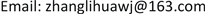﻿ 有限维模李超代数W⌒(n,m)的Z-阶化与其极大子代数 The Z-Graded and the Maximum Subalgebra of the Finite-Dimensional Modular Lie Superalgebra W⌒(n,m)

Pure Mathematics
Vol.07 No.06(2017), Article ID:22733,4 pages
10.12677/PM.2017.76063

The Z-Graded and the Maximum Subalgebra of the Finite-Dimensional Modular Lie Superalgebra $\stackrel{⌢}{W}\left(n,m\right)$

Lihua Zhang, Qi Cui

School of Mathematics and System Science, Shenyang Normal University, Shenyang LiaoningReceived: Nov. 1st, 2017; accepted: Nov. 15th, 2017; published: Nov. 21st, 2017ABSTRACT

This paper proves that the finite-dimensional modular Lie superalgebra $\stackrel{⌢}{W}\left(n,m\right)$ is Z-graded, and obtains a maximum subalgebra ${\stackrel{⌢}{W}}_{\left[0\right]}$ of it.

Keywords:Modular Lie Superalgebra, Z-Graded, Maximum Subalgebra1. 引言

2. 有限维模李超代数 $\stackrel{⌢}{W}\left(n,m\right)$ 的Z-阶化

${\stackrel{⌢}{W}}_{i}={\text{span}}_{F}\left\{{x}^{u}{y}^{\mu }{D}_{j}||u|+\delta \left(j,{I}_{2}\right)=i+1,u\in B\left(n\right),\mu \in H,j\in I\right\}$

$\left[{x}^{u}{y}^{\mu }{D}_{j},{x}^{v}{y}^{\lambda }{D}_{k}\right]={x}^{u}{y}^{\mu }{D}_{j}\left({x}^{v}{y}^{\lambda }\right){D}_{k}-{\left(-1\right)}^{|f||g|}{x}^{v}{y}^{\lambda }{D}_{k}\left({x}^{u}{y}^{\mu }\right){D}_{j}$ (1)

$j,k\in {I}_{1}$ ，对于 $f={x}^{u}{y}^{\mu }{D}_{j}\in {\stackrel{⌢}{W}}_{t}$ ，有：

$|u|+\delta \left(j,{I}_{2}\right)=|u|=t+1$

$|v|+\delta \left(k,{I}_{2}\right)=|v|=m+1$

${x}^{u}{x}^{v-〈j〉}{y}^{\mu }{y}^{\lambda }{D}_{k}$ ，如果 $\left\{u\right\}\cap \left\{v-\left\{j\right\}\right\}\ne \varnothing$ ，那么 ${x}^{u}{x}^{v-〈j〉}{y}^{\mu }{y}^{\lambda }{D}_{k}=0$ ，否则，因 $j\in {I}_{1}$ ，所以：

$|u|+|v-〈j〉|+\delta \left(j,{I}_{2}\right)=|u|+|v-〈j〉|=t+1+m+1-1=t+m+1$

${x}^{u}{y}^{\mu }{x}^{v-〈j〉}{y}^{\lambda }{D}_{k}\in {\stackrel{⌢}{W}}_{t+m}$ ，同理知 ${x}^{v}{x}^{u-〈k〉}{y}^{\lambda }{y}^{\mu }{D}_{j}\in {\stackrel{⌢}{W}}_{t+m}$ ，进而 $\left[{x}^{u}{y}^{\mu }{D}_{j},{x}^{v}{y}^{\lambda }{D}_{k}\right]\in {\stackrel{⌢}{W}}_{t+m}$ ，因此，当 $j,k\in {I}_{1}$ 时， $\left[{\stackrel{⌢}{W}}_{t},{\stackrel{⌢}{W}}_{m}\right]\subseteq {\stackrel{⌢}{W}}_{t+m}$

$j\in {I}_{2},\text{\hspace{0.17em}}k\in {I}_{2}$ ，对于 $f={x}^{u}{y}^{\mu }{D}_{j}\in {\stackrel{⌢}{W}}_{t}$ ，有：

$|u|+\delta \left(j,{I}_{2}\right)=|u|+1=t+1$

$|v|+\delta \left(k,{I}_{2}\right)=|v|+1=m+1$

${x}^{u}{y}^{\mu }{x}^{v}{y}^{\lambda }{D}_{k}$ ，如果 $\left\{u\right\}\cap \left\{v\right\}\ne \varnothing$ ，那么 ${x}^{u}{y}^{\mu }{x}^{v}{y}^{\lambda }{D}_{k}=0$ ，否则，因为 $k\in {I}_{2}$ ，所以有：

$|u|+|v|+\delta \left(j,{I}_{2}\right)=t+m+1$

${x}^{u}{y}^{\mu }{x}^{v}{y}^{\lambda }{D}_{k}\in {\stackrel{⌢}{W}}_{t+m}$ ，同理可知 ${x}^{v}{x}^{u}{y}^{\lambda }{y}^{\mu }{D}_{j}\in {\stackrel{⌢}{W}}_{t+m}$ ，进而 $\left[{x}^{u}{y}^{\mu }{D}_{j},{x}^{v}{y}^{\lambda }{D}_{k}\right]\in {\stackrel{⌢}{W}}_{t+m}$ ，因此当 $j,k\in {I}_{2}$ 时， $\left[{\stackrel{⌢}{W}}_{t},{\stackrel{⌢}{W}}_{m}\right]\subseteq {\stackrel{⌢}{W}}_{t+m}$

3. 有限维模李超代数 $\stackrel{⌢}{W}\left(n,m\right)$ 的极大子代数

$M$$\stackrel{⌢}{W}$ 的子代数，且 ${\stackrel{⌢}{W}}_{\left[0\right]}$ 真包含于 $M$ ，则存在 $x\in M$ ，使：

$x={x}_{-1}+y$

4. 结论

The Z-Graded and the Maximum Subalgebra of the Finite-Dimensional Modular Lie Superalgebra W(n,m)[J]. 理论数学, 2017, 07(06): 478-481. http://dx.doi.org/10.12677/PM.2017.76063

1. 1. 张永正, 刘文德. 模李超代数[M]. 北京: 科学出版社, 2004.

2. 2. 王璐, 张丽华. 有限维模李超代数 的单性[J]. 理论数学, 2014, 4: 247-250.

3. 3. Wang, X.L. and Liu, W.D. (2007) Filtered Lie Superalgebras of Odd Hamiltonian Type HO. Advances in Mathematics, 36, 710-720.

4. 4. 华秀英, 刘文德. 无限维Hamilton模李超代数的导子[J]. 数学研究与评论, 2007, 27(4):750-754.

5. 5. 徐晓宁. 有限维模李超代数 [D]: [博士学位论文]. 长春: 东北师范大学, 2010.

6. 6. 远继霞. Cartan型李超代数[D]: [博士学位论文]. 哈尔滨: 哈尔滨工业大学, 2011.

7. 7. 董艳琴. 广义Cartan型模李超代数[D]: [博士学位论文]. 长春: 东北师范大学, 2011.

8. 8. 徐晓宁, 王瑾. -型模李超代数的阶化[J]. 东北师范大学(自然科学版), 2016, 48(3): 1-4.